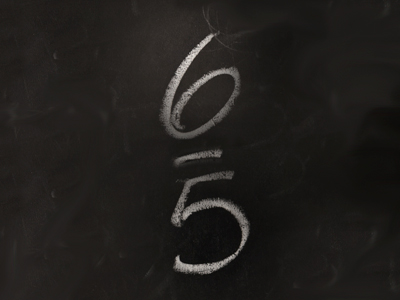65 is an improper fraction.

# Fractions 3

This Math quiz is called 'Fractions 3' and it has been written by teachers to help you if you are studying the subject at elementary school. Playing educational quizzes is an enjoyable way to learn if you are in the 3rd, 4th or 5th grade - aged 8 to 11.

It costs only \$12.50 per month to play this quiz and over 3,500 others that help you with your school work. You can subscribe on the page at Join Us

Fractions denote numbers that are less than a unit. One way of writing these 'partial' numbers is by using a decimal point. These are called decimal numbers. An example would be 2.5. 2.5 as a fraction would be written as 212. 212 is a mixed number. Another way to write it would be 52 which is an improper fraction. Proper fractions have numerators which are lower than their denominators.

See how much you have remembered from your classes by playing this quiz about proper and improper fractions, numerators and denominators.

1.
Which of these fractions is not equivalent to 13?
26
412
39
210
210 is the same as 15
2.
What would 314 be when converted to an improper fraction?
114
58
134
133
There are 44 in 1 unit so 3 units = 124
3.
Which of these is a proper fraction?
912
76
43
129
A proper fraction has a top number less than the bottom number
4.
Which fraction is more than 14?
28
312
620
25100
520 is equivalent to 14 so 620 is more
5.
What type of fraction is 65?
Mixed fraction
Equivalent fraction
Improper fraction
Unsuitable fraction
An improper fraction has a top number more than the bottom number
6.
Which fraction is less than 12?
51100
712
59
715
2 x 7 = 14 so 715 is slightly less than half
7.
What would 64 be when converted to a mixed number?
412
112
114
214
A mixed number is where there is a mix of a whole number and a fraction
8.
Which fraction is 25100 equivalent to?
14
18
12
15
100 ÷ 25 = 4
9.
Which fraction is one ninth?
18
19
16
112
19 is the same as 1 ÷ 9
10.
Which is the largest of these fractions?
39
912
35100
12
912 is larger because 9 is closer to 12 than 3 is to 9, 35 is to 100 and 1 is to 2
Author:  Amanda Swift